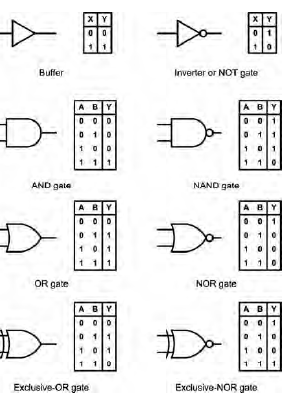# Digital fundamentals Logic gates

## Digital fundamentals Logic gatesDigital fundamentals

we introduce the basic building blocks of the digital logic systems found in modern aircraft.

We begin with a review of the different types of logic gates before moving on to explore their use

in several typical aircraft applications.

The chapter also provides a brief overview of coding systems and the logic arrangements that are used to generate and convert the codes that are used to represent numerical data.

The chapter provides an introduction to aircraft bus systems and concludes with a brief overview of

the architecture and principal constituents of simple computer systems.

Logic gates

Aircraft logic systems follow the same conventions

and standards as those used in other electronic

applications. In particular, the MIL/ANSI standard

logic symbols are invariably used and the logic elements

that they represent operate in exactly the same

way as those used in non-aircraft applications. MIL/

ANSI standard symbols for the most common logic

Gates

Buffers

Buffers do not affect the logical state of a digital

signal (i.e. a logic 1 input results in a logic 1 output

whereas a logic 0 input results in a logic 0 output).

Buffers are normally used to provide extra current

drive at the output but can also be used to regularize

the logic levels present at an interface.

Inverters are used to complement the logical

state (i.e. a logic 1 input results in a logic 0 output

and vice versa). Inverters also provide extra current

drive and, like buffers, are used in interfacing applications

where they provide a means of regularizing.

AND logic

AND gates will only produce a logic 1 output when

all inputs are simultaneously at logic 1. Any other

input combination results in a logic 0 output.

OR logic

OR gates will produce a logic 1 output whenever

any one, or more, inputs are at logic 1.

NAND logic

NAND (i.e. NOT-AND) gates will only produce a

logic 0 output when all inputs are simultaneously at

logic 1. Any other input combination will produce

a logic 1 output. A NAND gate, therefore, is nothing

more than an AND gate with its output inverted.

The circle shown at the output of the gate denotes this

inversion.

NOR logic

NOR (i.e. NOT-OR) gates will only produce a logic 1

output when all inputs are simultaneously at logic 0.

Any other input combination will produce a logic 0

output. A NOR gate, therefore, is simply an OR gate

with its output inverted. A circle is again used to indicate

inversion.

Exclusive-OR logic

Exclusive-OR gates will produce a logic 1 output

whenever either one of the two inputs is at logic 1 and

the other is at logic 0. Exclusive-OR gates produce a

logic 0 output whenever both inputs have the same

logical state (i.e. when both are at logic 0 or both are

at logic 1).